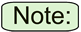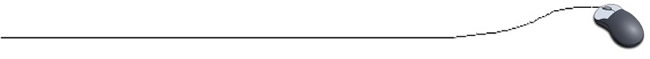Conditional Operator "?"
You can exchange simple if-else code for a single conditional operator, the ?. This conditional operator is a ternary operator (working on three values). The other logical (conditional) operators we have seen are called binary operators (working on two values.)This conditional operator is not commonly used. If you find it confusing, remember that there are other ways to accomplish the same task. It can always be replaced with a simple "if - else" statement. While this conditional operator may not be commonly used, it does have its useful situations, as we will see, so stay tuned.

FORMAT:         ans = (a>b) ? 25 : 45;

• The "condition" you will be testing, is in parentheses. (a > b)
• A question mark (the conditional operator). ?
• The value to use if the condition is TRUE. 25
• A colon. :
• The value to use if the condition is FALSE. 45
• A place to store the result. ans
 Equivalent to: if (a > b)     ans = 25; else     ans = 45;
The question mark helps the statement read as follows:
"Is a greater than b? If so, put 25 in ans. Otherwise, put 45 in ans."

Consider these comparison examples showing acceptable usage:
 if (age >= 18)    ticket = 12.50; else    ticket = 10.50; ticket = (age >=18) ? 12.5 : 10.5;

The ternary operator can also be used to determine what information to display.
 if (female.equals("married"))        System.out.print("Mrs. "); else    System.out.print("Ms. "); System.out.print( (female.equals("married")) ? "Mrs. " : "Ms. ");The primary job of this operator is to decide which value should be assigned to a variable.